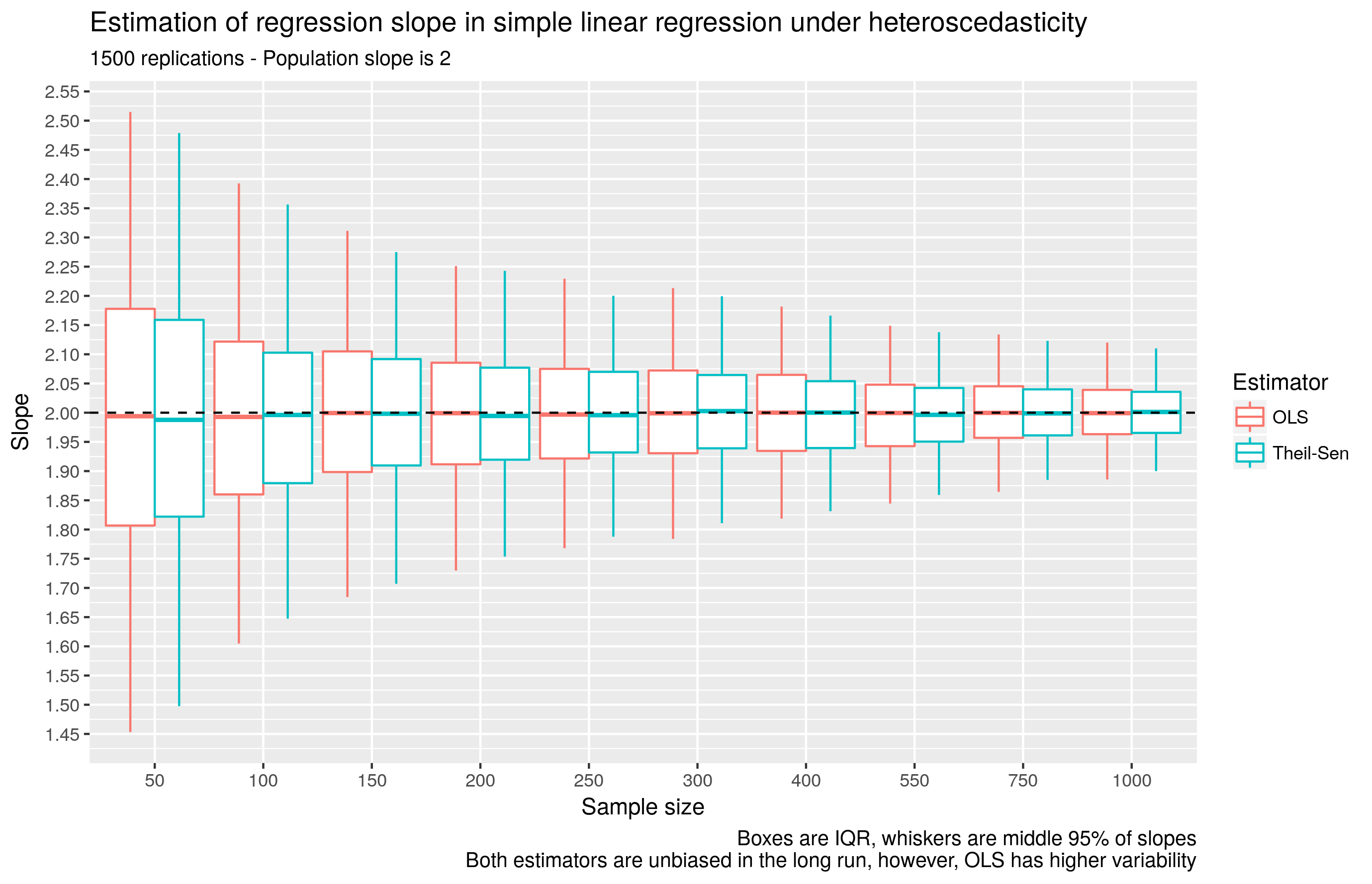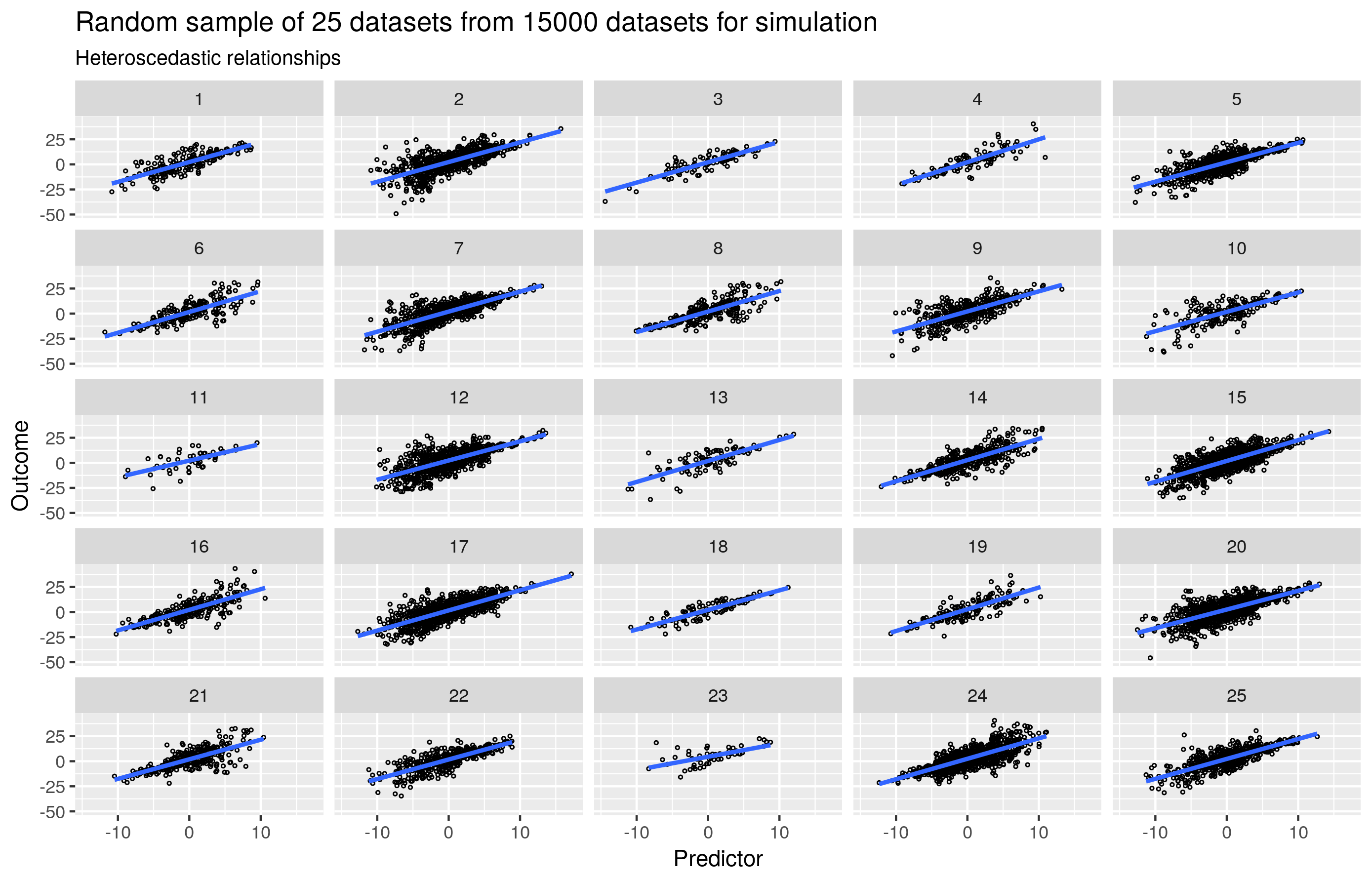# Theil-Sen regression in R

## 2017/09/21

TLDR: When performing a simple linear regression, if you have any concern about outliers or heterosedasticity, consider the Theil-Sen estimator.

A simple linear regression estimator that is not commonly used or taught in the social sciences is the Theil-Sen estimator. This is a shame given that this estimator is very intuitive, once you know what a slope means. Three steps:

• Plot a line between all the points in your data
• Calculate the slope for each line
• The median slope is your regression slope

Calculating the slope this way happens to be quite robust. And when the errors are normally distributed and you have no outliers, the slope is very similar to OLS.1

There are several methods to obtain the intercept. It is reasonable to know what your software is doing if you care for the intercept in your regression. Theil-Sen regression is available in two R packages I know of: WRS2 and mblm.

mblm includes a modification to Theil’s original method that has a higher breakdown point (more robust).3 This modification is the default method.

WRS contains two functions for Theil-Sen regression: Theil’s original method in the tsreg function, and a modification for small samples when there are tied values in the outcome in the tshdreg function.

Re my comment at the top regarding Theil-Sen for simple linear regression when there are concerns about outliers and heteroskedasticity, see Dietz4 and Wilcox5 below.

I conducted a toy simulation to see how Theil-Sen competes with OLS under heteroskedasticity; It is the more efficient estimator.1. Wilcox, R. R. (1998). A note on the Theil-Sen regression estimator when the regressor is random and the error term is heteroscedastic. Biometrical Journal, 40(3), 261–268. doi: 10.1002/(SICI)1521-4036(199807)40:3<261::AID-BIMJ261>3.0.CO;2-V ↩︎

2. Wilcox Robust Statistics - Rand Wilcox’s collection of robust methods. It is not available on CRAN, as CRAN requires proper documentation for all functions. This is a good set of installation instructions - https://web.archive.org/web/20170712140359/http://www.nicebread.de/installation-of-wrs-package-wilcox-robust-statistics/↩︎

3. Siegel, A. F. (1982). Robust regression using repeated medians. Biometrika, 69(1), 242–244. https://doi.org/10.1093/biomet/69.1.242 ↩︎

4. Dietz, E. J. (1987). A comparison of robust estimators in simple linear regression. Communications in Statistics - Simulation and Computation, 16(4), 1209–1227. https://doi.org/10.1080/03610918708812645 ↩︎

5. Wilcox, R. R. (1998). A note on the Theil-Sen regression estimator when the regressor is random and the error term is heteroscedastic. Biometrical Journal, 40(3), 261–268. ↩︎